Many business activities generate data that can be thought of as random. An example described in the textbook is the servicing of cars at an oil change shop. Each car entering the shop can be considered an experiment with random outcomes. A variable of interest in this experiment could be the amount of time necessary to service the car. Service time will vary randomly with each car.

Don't use plagiarized sources. Get Your Custom Essay on
Get an essay WRITTEN FOR YOU, Plagiarism free, and by an EXPERT!

We can often capture the most relevant characteristics of a stochastic process with a simple probability distribution model. We can then analyze the model to make predictions and drive decisions. For instance, we could estimate the number of technicians the oil change shop needs to service demand on a Saturday afternoon. Discuss the following:

• What is a random variable?
• How would you differentiate a discrete from a continuous random variable?

A laptop manufacturing company has implemented a 2-step process to test the quality of each production batch. In the first step, a technician randomly selects 15 laptops from the batch and determines whether they meet specifications. The batch is considered acceptable provided no more than 1 laptop fails to meet specifications. Otherwise, the entire batch must be tested in the second step. Historical data shows that 95% of the laptops produced adhere to specifications. Discuss the following:

• What are the 4 characteristics of a binomial experiment?
• Can we use a binomial distribution to model this process?
• What is the probability that the entire batch unnecessarily has to be tested if in fact 95% of its laptops conform to specifications? (Hint: Use Excel’s =BINOMDIST() function to find the probability.)
• What is the probability that the batch is incorrectly accepted if only 75% of its laptops conform to specifications?

Week 3 Discussion

A random variable in statistics is a variable whose value is dependent on the numerical outcome of a random experiment or event, meaning it is not fixed but can take any value of all possible values in that experiment or event. Hence, a random variable can be a set of possible values in a random experiment. The difference between a discrete random variable from a continuous random variable is that discrete variables have several countable possible values obtained through counting. In contrast, continuous random variables are sets of possible values in a random experiment or event obtained through measuring (Boundless.com, 2017). For instance, if the values of Sample Y are countable, such as counting the number of theft cases taking place in an area between 9:00 pm-12:00, then Y becomes a discrete random variable. However, if the values of Sample Y are uncountable, such as identifying the height of children or IQ of students in a class, showing a take of random or any figures, then Y becomes a continuous random variable.

The four salient characteristics of a binomial experiment include having a fixed sample size or identical trials (n): in each test or process, results are one of the two possible outcomes, which are success or failure: success probability (p) is regularly similar in each trial: and finally, the trials (n) remain independent. Regarding the case example, a binomial distribution can be used. Primarily, n = 15, which is a satisfying assumption: secondly, the only two possible outcomes include having the laptops either meet the specified specifications (success) or fail to meet them (failure), hence satisfies the characteristics. Thirdly, the success probability is fixed, where the laptops must adhere to the 95% or 0.95 specifications, showing a regularly similar success probability in the two trails. Finally, having satisfied other characteristics of a binomial experiment, we can assume that the trails are independent.

When calculating the probability if 95% of the laptops conform to specifications means that the batch would be considered acceptable if not more than 1 laptop fails. Using X (laptops not met specifications) and n=15, p= 1-0.95= 0.05. P (if more than 1 laptop does not meet the specifications) = P(X>1) “= BINOMDIST (1, 15, 0.05, 1)” = 0.829047. P(X>1) = 1-0.829047= 0.170953. The calculation with 75% is similar, hence, probability to conform = 0.75 and not conform = 1-0.75 = 0.25. Thus, P (not more than 1 laptop not conform) = P(X>1)  “= BINOMDIST (1, 15, 0.25, 1)” = 0.080181.

ReferenceOrder NOW For A 10% Discount!
Pages (550 words)
Approximate price: -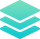Plagiarism Free Papers

All our papers are original and written from scratch. We will email you a plagiarism report alongside your completed paper once done.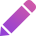Free Revisions

All papers are submitted ahead of time. We do this to allow you time to point out any area you would need revision on, and help you for free.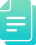Title-page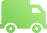BibliographyOriginality & Security

At Homework Sharks, we take confidentiality seriously and all your personal information is stored safely and do not share it with third parties for any reasons whatsoever. Our work is original and we send plagiarism reports alongside every paper.Our agents are online 24/7. Feel free to contact us through email or talk to our live agents.

Try it now!

## Calculate the price of your order

We'll send you the first draft for approval by at
Total price:
\$0.00

How it works?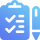Fill in the order form and provide all details of your assignment.Proceed with the payment

Choose the payment system that suits you most.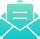Our Services

We work around the clock to see best customer experience.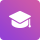## Flexible Pricing

Our prces are pocket friendly and you can do partial payments. When that is not enough, we have a free enquiry service.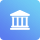When you need to elaborate something further to your writer, we provide that button.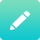Paper Submission

We take deadlines seriously and our papers are submitted ahead of time. We are happy to assist you in case of any adjustments needed.Customer Feedback

Your feedback, good or bad is of great concern to us and we take it very seriously. We are, therefore, constantly adjusting our policies to ensure best customer/writer experience.